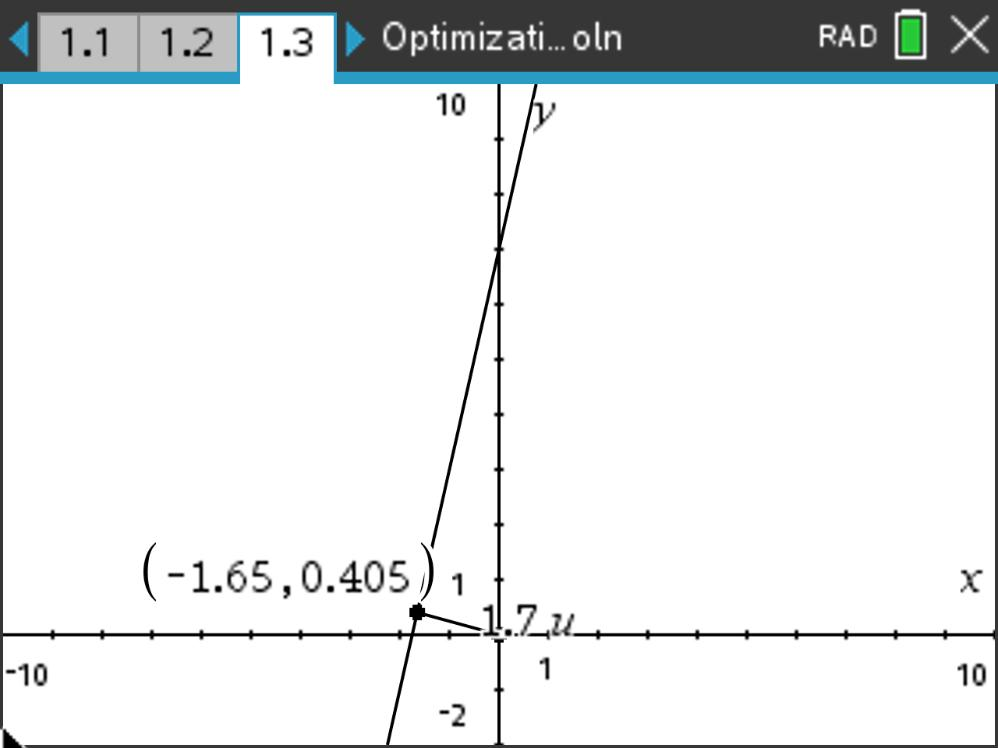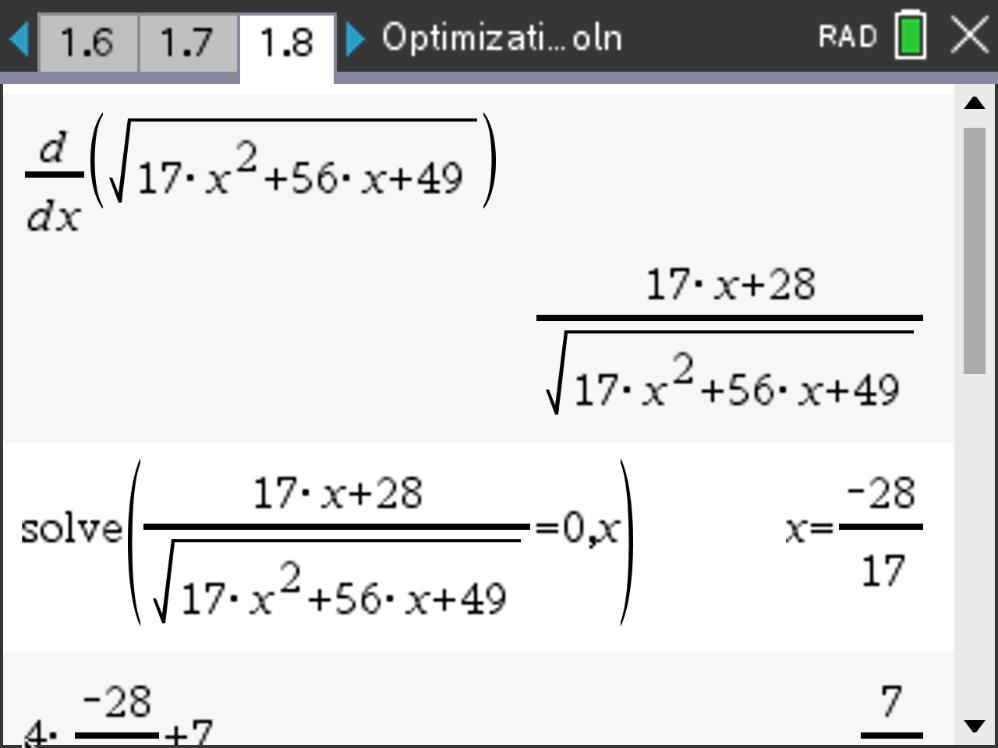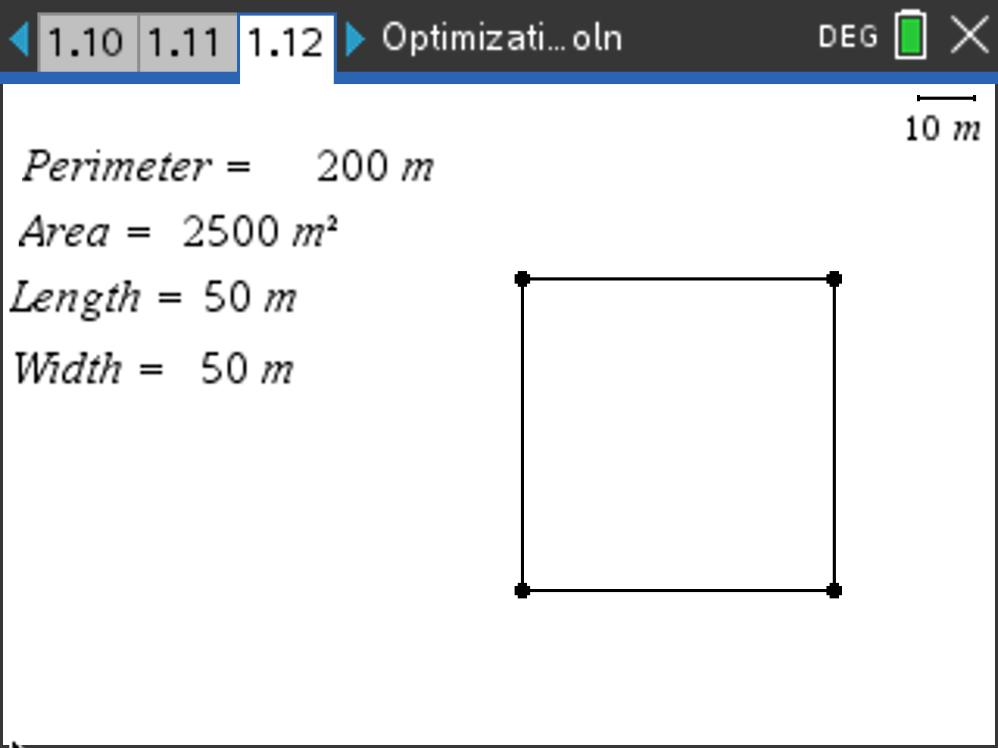### Mathematics lessons for IB® Diploma Programme

Analysis and Approaches | Calculus

# Optimization

Students learn how to use the second derivative test to find maxima and minima in word problems and solve optimization in parametric functions.

## Key Steps## Step 1

In Problem 1, students investigate optimization of distance and area problems. They begin by graphing a linear equation and constructing a point on the line. This point is then connected to the origin, and students seek to find the coordinates of the point (x, y) that minimizes the length of this segment.

Here, the linear equation acts as the constraint, which students may use to rewrite the distance function in one variable.## Step 2

Students then find the first derivative, and apply the second derivative test to find the minimum at the critical value of x. They substitute the value of x into the original linear equation to determine the y-coordinate.

Students can confirm this answer by using the fmin command to obtain the x-value of the minimum point.## Step 3

Also in Problem 1, students will consider a rectangle with a fixed perimeter of 200 m, seeking to maximize its area. In a manner similar to that above, students maximize the function A = l w with the constraint 2l + 2w = 200.